Courses

# Test: Control System- 1

## 25 Questions MCQ Test Basic Electronics Engineering for SSC JE (Technical) | Test: Control System- 1

Description
This mock test of Test: Control System- 1 for Electrical Engineering (EE) helps you for every Electrical Engineering (EE) entrance exam. This contains 25 Multiple Choice Questions for Electrical Engineering (EE) Test: Control System- 1 (mcq) to study with solutions a complete question bank. The solved questions answers in this Test: Control System- 1 quiz give you a good mix of easy questions and tough questions. Electrical Engineering (EE) students definitely take this Test: Control System- 1 exercise for a better result in the exam. You can find other Test: Control System- 1 extra questions, long questions & short questions for Electrical Engineering (EE) on EduRev as well by searching above.
QUESTION: 1

Solution:
QUESTION: 2

Solution:
QUESTION: 3

### What is the characteristic of a good control system ?

Solution:
QUESTION: 4

The transfer function of a linear system is the

Solution:
QUESTION: 5

If the open-loop transfer function is a ratio of a numerator polynomial of degree 'm' and a denominator polynomial of degree 'n' then the integer (n-m) represents the number of

Solution:
QUESTION: 6

Despite the presence of negative feedback, control system still have problems of instability  because the

Solution:
QUESTION: 7

Consider the following statements in connection with the feedback of control systems:

1. Feedback can improve stability or be harmful to stability if it is not properly applied.

2. Feedback can always improve stability

.3. In many situations the feedback can reduce the effect of noise and disturbance on system performance.

4. In general the sensitivity of the system gain of a feedback system to a parameter variatio depends on, where the parameter is located.

Which of the above statements are correct ?

Solution:
QUESTION: 8

Consider the following statements:

1. The effect of feedback is to reduce the system error.

2. Feedback increases the gain of the system in one frequency range but decreases in another.

3. Feedback can cause a system that is originally stable to become unstable.

Which of these statements are correct ?

Solution:
QUESTION: 9

Consider the following statements:

Feedback in control system can be used

1. to reduce the sensitivity of the system to parameter variations and disturbances.

2. to change time constant of the system.

3. to increase loop gain of the system

Which of the statement given above are correct?

Solution:
QUESTION: 10

With negative feedback in a closed loop control system, the system sensitivity to parameter variations

Solution:
QUESTION: 11

In a control system, the use of negative feedback

Solution:
QUESTION: 12

The transfer function of a system is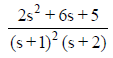The characteristic equation of the system is

Solution:
QUESTION: 13

Which one of the following identity is correct one?

Solution:
QUESTION: 14

The transfer function from d(s) to y(s) is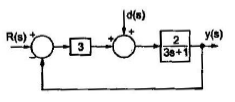Solution:
QUESTION: 15

For what value of K, are the two block diagrams as shown below equivalent ?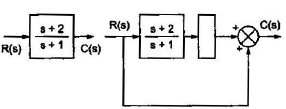Solution:
QUESTION: 16

In the signal flow graph of the figure is y/x equals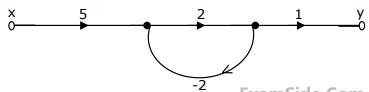Solution:
QUESTION: 17

For the signal flow diagram shown in the given figure, the transmittance between x2 and x1 is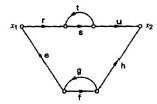Solution:
QUESTION: 18

When a human being tries to approach an object, his brain acts as

Solution:
QUESTION: 19

Consider the loop transfer function

G(s)H(s) =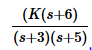In the roots locus diagram the controid will be located at

Solution:
QUESTION: 20

Consider the following statements:

In root-locus plot, the breakaway points

1. Ned not always be on real axis alone

2. Must lie on the root loci

3. Must lie between 0 and – 1

Which of these statements are correct ?

Solution:
QUESTION: 21

For a unity negative feedback control system, the open loop transfer function is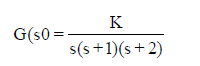The root-locus plot of the system is

Solution:
QUESTION: 22

First column elements of the Routh's tabulation are 3, 5 -3/4, 1/2, 2. It means that there

Solution:
QUESTION: 23

The response c(t) of a system is described by te differential equation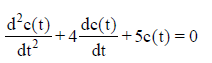The system response is

Solution:
QUESTION: 24

The polar plot of a transfer function passes through the critical point (–1.0). The gain margin is

Solution:
QUESTION: 25

If the transfer function of a phase lead compensator is (s+ a)/ (s+b) and that of a lag compensator is (s + p)/(s+q), then Which one of the following sets of condition must be satisfied ?

Solution: